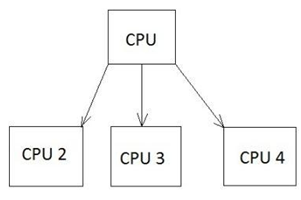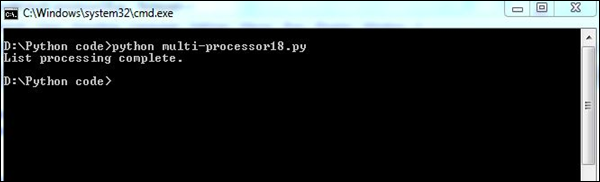# Python Forensics - Multiprocessing Support

Forensic specialists normally find it difficult to apply digital solutions to analyze the mountains of digital evidence in common crimes. Most digital investigation tools are single threaded and they can execute only one command at a time.

In this chapter, we will focus on the multiprocessing capabilities of Python, which can relate to the common forensic challenges.

## Multiprocessing

Multiprocessing is defined as the computer system's ability to support more than one process. The operating systems that support multiprocessing enable several programs to run concurrently.

There are various types of multiprocessing such as symmetric and asymmetric processing. The following diagram refers to a symmetric multiprocessing system which is usually followed in forensic investigation.### Example

The following code shows how different processes are listed internally in Python programming.

```import random
import multiprocessing

def list_append(count, id, out_list):
#appends the count of number of processes which takes place at a time
for i in range(count):
out_list.append(random.random())

if __name__ == "__main__":
size = 999
procs = 2
# Create a list of jobs and then iterate through
# the number of processes appending each process to
# the job list
jobs = []

for i in range(0, procs):
out_list = list() #list of processes
process1 = multiprocessing.Process(
target = list_append, args = (size, i, out_list))

# appends the list of processes
jobs.append(process)

# Calculate the random number of processes
for j in jobs:
j.start()  #initiate the process

# After the processes have finished execution
for j in jobs:
j.join()
print "List processing complete."
```

Here, the function list_append() helps in listing the set of processes in the system.

### Output

Our code will produce the following output −